# Configuring Dynamics 365 Currency Fields in Power BI

### Colin Maitland, 10 October 2019

In this article, I demonstrate the data type conversion that should be applied to Dynamics 365 Currency fields in Power BI; ie. to Fixed Decimal Number instead of to Decimal Number.

In Microsoft Dynamics 365, “Currency” fields are stored in the underlying SQL Server database using the SQL Server Money data type. The Money data type is a Fixed Decimal Number data type and is similar to but not the same size as the Decimal(19, 4) Fixed Decimal Number data type, which is one byte larger and so can store a broader range of values.

In Power BI, Dynamics 365 Currency fields are converted automatically to the Power BI Decimal Number data type. The “1.2” icon before the column names in the Power Query Editor indicates this data type.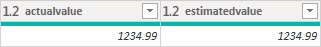In Power BI, Decimal Number fields are stored as Double-Precision Floating-Point fields; i.e. the Float(53) data type. This data type uses scientific notation.

SQL Server Decimal data type fields, such as Decimal(19, 4) and Decimal(38, 4), are Fixed Decimal Number fields while Power BI Decimal Number fields are Floating Point fields.

It is important to be aware of this because calculations and comparisons using the Floating Point data type are not as precise as those using the Fixed Decimal Number data type; e.g. a Floating Point value of 99.999999999999999 is not the same as a Fixed Decimal Number value of 99.9999. When working with Currency values, preciseness and comparability are very important.

The following image show the results of a simple Total GST (Goods and Services Tax) calculation performed against 100 records using the Power BI Decimal Number versus Fixed Decimal Number data types. The result calculated using the Decimal Number data type is not precise to 4 decimal places, and when compared with the result calculated using the Fixed Decimal Number data type is not the same.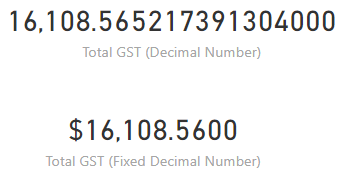In Power BI, Currency data type fields retrieved from Dynamics 365 should be converted to the Fixed Decimal Number data type. You can do this with Power Query using the Data Type options on the Transform tab in the Power Query Editor.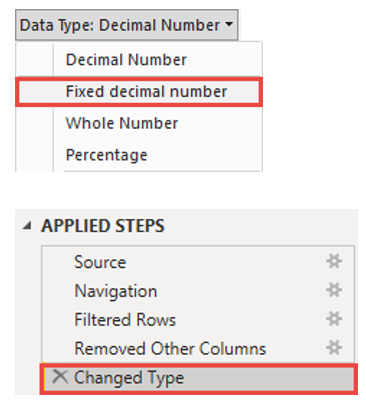The “\$” icon before the column names in the Power Query Editor indicate the change of data type and indicates that this data type is a Currency specific data type.

Page Break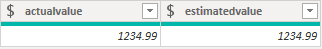Power BI Fixed Decimal Number values are automatically formatted and displayed in the Data and Report areas of Power BI as Currency formatted values.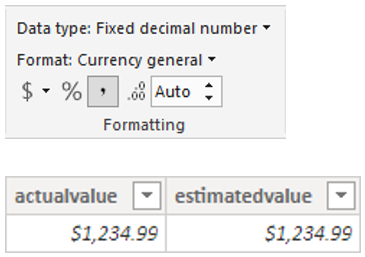You have the option of changing the format for your specific use as shown in this example where the format has been changed to display 0 decimal places, resulting in an automatic rounding of the displayed value, without any effect on the underlying stored value used by calculations.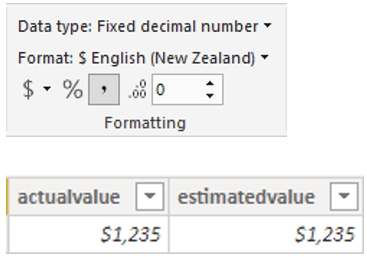Finally, changing the format of a number in Power BI does not change its data type. Therefore, simply changing the Format of a Decimal Number data type field to Currency does not change its data type; it remains a Floating-Point field rather than a Fixed Decimal Number field.